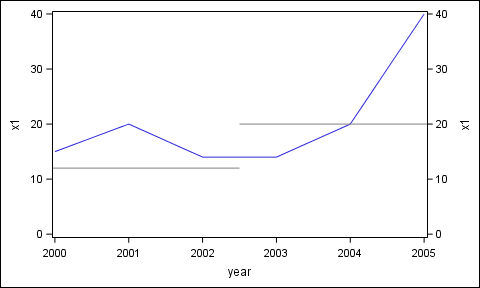## "Broken" reference line (different lines for different periods)

How can I draw a "broken" reference line, where there are different reference values for different sub-periods? For example:

data have;

input year x1 ref_val;

datalines;

2000 15 12

2001 20 12

2002 14 12

2003 14 20

2004 20 20

2005 40 20

;

run;

I've tried this code:

proc sgplot data=have;

series x=year y=x1;

refline ref_val/axis=y;

yaxis min=0;

run;

but this gives me two paralel lines for all observations, while I want  the reference line to be drawn at 12 only for the first three observations and then at 20 only for the last three ones.

Combining two series produces something closer to what I want but not exactly - there is still an unneccesary line joining 12 and 20:

proc sgplot data=have;

series x=year y=x1;

series x=year y=ref_val;

yaxis min=0;

run;

1 ACCEPTED SOLUTION

Accepted Solutions

## Re: "Broken" reference line (different lines for different periods)

You can use this annotate dataset and get the results.

data annoLine;

retain drawspace "datavalue" linecolor "blue";

input function \$ x1 y1 x2 y2;

datalines;

line 2000 12 2002 12

line 2003 20 2005 20

;

run;

proc sgplot data=have sganno=annoLine;

series x=year y=x1;

yaxis min=0;

run;

9 REPLIES 9

## Re: "Broken" reference line (different lines for different periods)

@chris2377 wrote:

I want  the reference line to be drawn at 12 only for the first three observations and then at 20 only for the last three ones.

If you only have a few observations, you might consider drawing 2 lines by annotating the graph

## Re: "Broken" reference line (different lines for different periods)

You can use this annotate dataset and get the results.

data annoLine;

retain drawspace "datavalue" linecolor "blue";

input function \$ x1 y1 x2 y2;

datalines;

line 2000 12 2002 12

line 2003 20 2005 20

;

run;

proc sgplot data=have sganno=annoLine;

series x=year y=x1;

yaxis min=0;

run;

## Re: "Broken" reference line (different lines for different periods)

Thanks. I've tried to avoid annotate but I see it's the best option

## Re: "Broken" reference line (different lines for different periods)

Annotate or some extra data manipulation. This may be close to what I think you're attempting:

``````data have;
input year year1 year2 x1 ref_val;
datalines;
2000 .  . 15  .
2001 .  . 20  .
2002 .  . 14  .
2003 .  . 14  .
2004 .  . 20  .
2005 .  . 40  .
. 2000  .  . 12
. 2001  .  . 12
. 2002  .  . 12
.  .  2003 . 20
.  .  2004 . 20
.  .  2005 . 20
;
run;

proc sgplot data=have;
series x=year y=x1;
series x=year1 y=ref_val;
series x=year2 y=ref_val;
yaxis min=0;
run;
``````

## Re: "Broken" reference line (different lines for different periods)

@ballardw

Thanks. Data manipulation is a nice alternative to annotate. Following the idea from your post, I've come up with a bit simpler code:

data have;

set have;

if year<2003 then refline1=12;

if year>2002 then refline2=20;

run;

proc sgplot data=have;

series x=year y=x1;

series x=year y=refline1;

series x=year y=refline2;

yaxis min=0;

run;Jay54
Meteorite | Level 14

## Re: "Broken" reference line (different lines for different periods)

SAS 9.4 SGPLOT includes the DROPLINE statement that is designed for just such a use case.  Also, for discrere axes, you can use the DiscreteOffset feature to extend the line half way between categories.

## Re: "Broken" reference line (different lines for different periods)

@Jay54

Thanks for the suggestion, but dropline still produces a line at 20 for all observations:

proc sgplot data=have;

series x=year y=x1;

yaxis min=0;

dropline x=year y=ref_val/dropto=y;

run;Jay54
Meteorite | Level 14

## Re: "Broken" reference line (different lines for different periods)

See codee below.  I have added specific values for the DropLine, but they can come from data.

Note, one of the dropto has to be to the Y2 axis, so there needs to be a Y2 axis.  The 2nd series plot is added to create the Y2 axis, but the line thickness is set to zero.  Y and Y2 have same extents.

data have;
input year x1 ref_val;
datalines;
2000 15 12
2001 20 12
2002 14 12
2003 14 20
2004 20 20
2005 40 20
;
run;

proc sgplot data=have;
series x=year y=x1;
refline ref_val/axis=y;
yaxis min=0;
run;

proc sgplot data=have;
series x=year y=x1;
series x=year y=x1 / y2axis lineattrs=(thickness=0);
dropline x=2002.5 y=12 / dropto=y;
dropline x=2002.5 y=20 / dropto=y y2axis;
yaxis min=0;

y2axis min=0;
run;## Re: "Broken" reference line (different lines for different periods)

@Jay54

Thanks a lot. A bit trickier than I thought but gives what I wanted.

To sum up for future readers - 3 possible ways of dealing with the issue:

Solution I - dropline

proc sgplot data=have;

series x=year y=x1;

series x=year y=x1 / y2axis lineattrs=(thickness=0);

dropline x=2002.5 y=12 / dropto=y;

dropline x=2002.5 y=20 / dropto=y y2axis;

yaxis min=0;

y2axis min=0;

run;

Solution II - data manipulation

data have;

set have;

if year<2003 then refline1=12;

if year>2002 then refline2=20;

run;

proc sgplot data=have;

series x=year y=x1;

series x=year y=refline1;

series x=year y=refline2;

yaxis min=0;

run;

Solution III - annotate

data annoLine;

retain drawspace "datavalue" linecolor "blue";

input function \$ x1 y1 x2 y2;

datalines;

line 2000 12 2002 12

line 2003 20 2005 20

;

run;

proc sgplot data=have sganno=annoLine;

series x=year y=x1;

yaxis min=0;

run;

Discussion stats
• 9 replies
• 1456 views
• 1 like
• 4 in conversation